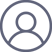龚明2022-5-25 12:42

Any phase-space configuration $(p, q)$ of a system enclosed in a finite volume will be repated as accurately as one whshes after a finite (be it possibly very long) interval of time.

In mathematics and physics, the Poincaré recurrence theorem states that certain dynamical systems will, after a sufficiently long but finite time, return to a state arbitrarily close to (for continuous state systems), or exactly the same as (for discrete state systems), their initial state.

\begin{equation} \mathcal{K} = \{|n\rangle | \quad  n=0, 1, 2, \cdots. \} \end{equation}

\begin{equation} |\Psi(t)\rangle  = \sum_{n=0}^\infty r_n e^{i\phi_n -iE_n t} |n\rangle. \end{equation}

\begin{equation}|| \Psi(T) - \Psi(0)|| = 2\sum_{n=0}^\infty r_n^2 (1 - \cos(E_n t)). \end{equation}我们要证明存在$T$，使得\begin{equation} 2\sum_{n=0}^\infty r_n^2 (1 - \cos(E_n t)) \le \epsilon, \quad \epsilon \rightarrow 0^+. \end{equation}注意这里有无穷多能级，所以不好处理。我们需要做一个截断，比如我们假设存在$N$, 使得\begin{equation}2\sum_{n=N+1}^\infty r_n^2(1 - \cos(E_n t))  \le 4\sum_{n=N+1}^\infty r_n^2  \le \epsilon/3. \end{equation} 这一点总是可以做到的，因为$\sum_{n=0}^\infty r_n^2 = 1$是归一的。

1. $E_n$都是有理数，假设$E_n = q_n/p_n$, 那么可以定义时间$T =2 \pi \prod_{j} p_j$。此时，系统它格地回复到初始状态。如果这个近似可以足够精确，则这个结论也是成立的。

2. $E_n$不完全是有理数，此时我们可以假设（这个解非唯一, 而且可以无限逼近）

\begin{equation}E_n = {q_n + \kappa_n \over K}, \quad  |\kappa_n| < q \sqrt{\epsilon}. \end{equation}

取$T =2\pi K$，则\begin{equation} \sum_{n=0}^N 2r_n^2 (1 - \cos(E_n2 \pi K)) \le \sum_{n=0}^N r_n^2 \kappa_n^2  \le  4\pi^2 q^2 \epsilon. \end{equation}

由于$E_n$可以用有理数无限逼近，这个要求总是可以得到满足的---这个证明的细节，我们不讨论。它要求（无理数的性质）

\begin{equation} |E_n - {q_n \over K}| < {q\sqrt{\epsilon} \over K}. \end{equation}

它可以用构造法得到。对于任意一个趋于零的正数$\delta$（$\delta \rightarrow 0^+$）, 以及任意一组离散的值$E_n$,  $0 \le n\le N$，总可以找到合适的整数$a_n$和$b_n$, 使得$|E_n - a_n/b_n| < \delta$成立。如果这样，可以取$K = \prod_n b_n$, $q_n = a_n K/b_n$，以及$\delta = q\sqrt{ \epsilon}/K$即可（此处证明不完全，需要仔细讨论）。最后选择合适的$q$即可得到量子回归定理。

\begin{equation} \sum_{n=0}^N |r_n e^{iE_n t} - r_n|^2  < {\epsilon \over 3}. \end{equation} 这个结论数学家早就得到了； 但如上所示，它有简单和直观的图像。

\begin{equation} ||\Psi(T) - \Psi(0)|| = \sqrt{{A \over \pi} }\int dx \exp(-A x^2) (1 - \cos(x T)) = 1 - \exp(-{T^2 \over 4A}).  \end{equation}

\begin{equation} I = \sum_{j=1}^N \ln(A + B \cos(q j)).\end{equation}

\begin{equation} I \simeq  {N \over 2\pi} \int_0^{2\pi} \ln(A + B \cos(\theta)) d\theta.\end{equation}

\begin{equation} I = \int f(x) dx = a \sum_i f(x = ia). \end{equation}

2017年第一次“证明”这个结论；2022年5月24整理。